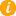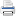Math K-Plus

# Practice 3rd Grade Multiplication Calculator

TestQuizQuiz Results• Option 2. Solve a calculator generated math problem. First press the NEW PROBLEM button. Next enter your answer, in to the calculator. Lastly, press the ADD button and then compare your answer with the calculator's.

Field Name Multiply Values
Multiplicand: You can enter up to 2 digits
Multiplier: You can enter only 2 digits
×After learning Third Grade Multiplication Calculator, you can move to the next step in multiplication math: Fourth Grade Multiplication Calculator.

+97
× +17
——————

97
×  17
——————

4
97
×  17
——————
9

64
97
×  17
——————
79

97
×  17
——————
679

97
×  17
——————
679
7

97
×  17
——————
679
97

97
×  17
——————
679
97

97
×  17
——————
679
97
——————
+1649